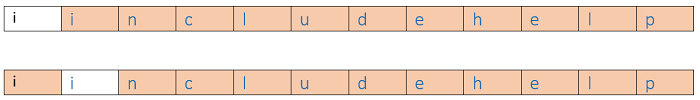# Jumbled Strings

Jumbled Strings: In this article, we are going to see a recursive problem (Jumbled Strings) and its solution that can be featured in any interview rounds.
Submitted by Radib Kar, on June 07, 2020

Problem statement:

You are provided an input string S and the string "includehelp". You need to figure out all possible subsequences "includehelp" in the string S? Find out the number of ways in which the subsequence "includehelp" can be formed from the string S.

Input:

Input is the string s

Output:

Corresponding to each test case, print in a new line, a number denoting the number of ways in which we can form the subsequence "includehelp". Since output can be very large find the answer modulo 1000000007.

Constraints:

Input String contains only lowercase English Letters and string length is 5000 at maximum.

Example:

```Input:
includehelp

Output:
1

Explanation:
There is only one instances of "includehelp"
in the above input string.

Input:
iincludehelp

Output:
2

Explanation:
There is two instances of "includehelp"
in the above input string.```

Solution Approach:

```Let the input string be, s and t="includehelp"

This problem can be solved by recursion.

So we have,
string s: the input string
string t: the second string ("includehelp")
starts  : start point of string s
srartt  : start point of string t, ("includehelp")

m: length of input string
11: length of string t,"includehelp"

MOD: 1000000009
```

Now, how can we generate a recursive relation?

Say starts=i where 0<=i<m & startt=j where 0<=j<11

Say,

1. s[starts]=t[startt] that means both have same character,
Now we have two options,
1. Check for starts+1, startt+1 which means we are looking for the same occurrence, we want to check for other characters as well.
2. Check for starts+1, startt which means we are looking for another different occurrence.
2. s[starts]!=t[startt]
Now we have only one option which is check for starts+1, startt as we need to look for different occurrence only.
```Function:
jumbleString(string s,string t,int starts,int startt,int m)
// enter substring is matched
if startt==11
return 1;

// enter string has been searched with out match
if starts==m
return 0;

if(s[starts]!=t[startt])
//only one option as we discussed
return jumbleString(s,t,starts+1,startt,m)%MOD;
else
// both the options as we discussed
return (jumbleString(s,t,starts+1,startt+1,m)%MOD +
jumbleString(s,t,starts+1,startt,m)%MOD)%MOD
```

The above recursion will generate many overlapping subproblems and hence we need to use dynamic programming.

Let's convert the recursion to DP.

• Step 1: initialize DP table,
```int dp[m+1];
```
• Step 2: convert step1 of recursive function,
```for i=0 to 11
dp[i]=0;
```
• Step 3: convert step 2 of recursive function,
```for i=0 to m
dp[i]=1;
```
• Step 4: Fill the DP table which is similar to step3 of the recursion function,
```for i=1 to m
for j=1 to 11
if s[i-1]==t[j-1]
dp[i][j]=(dp[i-1][j]+dp[i-1][j-1])%MOD
else
dp[i][j]=dp[i-1][j]
end for
end for
```
• Step5: return dp[m] which is the result.

The above DP technique is known as the tabulation process. We can introduce memorization as well, known as the top-down approach. Where we store every computed subproblem and while computing first we look up our DP table whether sub-problem is already solved or not. Check the below top-down implementation for the above problem.

C++ Implementation:

```#include <bits/stdc++.h>
using namespace std;

int dp;

int jumbled(string s1, string s2, int i, int j, int n, int m)
{

if (j == m) {
return 1;
}
if (i == n && j != m)
return 0;

if (dp[i][j] != -1)
return dp[i][j];

if (s1[i] == s2[j]) {
dp[i][j] = jumbled(s1, s2, i + 1, j + 1, n, m) + jumbled(s1, s2, i + 1, j, n, m);
}
else {
dp[i][j] = jumbled(s1, s2, i + 1, j, n, m);
}

return dp[i][j];
}

int main()
{
int n;

string s2 = "includehelp";

string s1;
cout << "Input string: ";
cin >> s1;

n = s1.length();

for (int i = 0; i < n; i++) {
for (int j = 0; j < 11; j++)
dp[i][j] = -1;
}

cout << "We can jumble " << jumbled(s1, s2, 0, 0, n, 11) << " of ways." << endl;

return 0;
}
```

Output:

```Input string: iincludehelp
We can jumble 2 of ways.
```

What's New

Top Interview Coding Problems/Challenges!

Languages: » C » C++ » C++ STL » Java » Data Structure » C#.Net » Android » Kotlin » SQL
Web Technologies: » PHP » Python » JavaScript » CSS » Ajax » Node.js » Web programming/HTML
Solved programs: » C » C++ » DS » Java » C#
Aptitude que. & ans.: » C » C++ » Java » DBMS
Interview que. & ans.: » C » Embedded C » Java » SEO » HR
CS Subjects: » CS Basics » O.S. » Networks » DBMS » Embedded Systems » Cloud Computing
» Machine learning » CS Organizations » Linux » DOS
More: » Articles » Puzzles » News/Updates